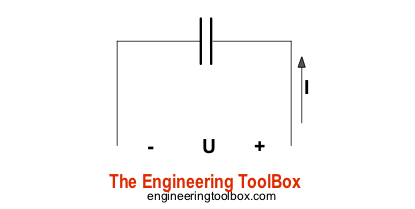Engineering ToolBox - Resources, Tools and Basic Information for Engineering and Design of Technical Applications!

# Capacitors - Stored Energy

## Potential power and energy stored in capacitors.### Capacitor - Energy Stored

The work done in establishing an electric field in a capacitor, and hence the amount of energy stored - can be expressed as

W = 1/2 C U2                     (1)

where

W = energy stored - or work done in establishing the electric field (joules, J)

C = capacitance (farad, F, µF)

U = potential difference (voltage, V)

### Capacitor - Power Generated

Since power is energy dissipated in time - the potential power generated by a capacitor can be expressed as

P = dW / dt                    (2)

where

P = potential power (watts, W)

dt = dissipation time (s)

### Example - Capacitor, energy stored and power generated

The energy stored in a 10 μF capacitor charged to 230 V can be calculated as

W = 1/2 (10 10-6 F) (230 V)2

= 0.26 J

in theory - if this energy is dissipated within 5 μs the potential power generated can be calculated as

P = (0.26 Joules) / (5 10-6 s)

= 52000 W

= 52 kW

Be aware that in any real circuit, discharge starts at a peak value and declines. The energy dissipated is a very rough average power over the discharge pulse.

### Capacitor - Time to Discharge at Constant Power Load

The time to discharge a capacitor at constant power load can be expressed as

dt = 1/2 C (Us2 - Uf2) / P               (3)

where

dt = discharge time (s)

Us = start voltage (V)

Uf = final voltage (V)

## Related Topics

• Environment - Climate, meteorology, sun, wind and environmental related engineering resources.
• Electrical - Electrical units, amps and electrical wiring, wire gauge and AWG, electrical formulas and motors.

## Engineering ToolBox - SketchUp Extension - Online 3D modeling!

Add standard and customized parametric components - like flange beams, lumbers, piping, stairs and more - to your Sketchup model with the Engineering ToolBox - SketchUp Extension - enabled for use with the amazing, fun and free SketchUp Make and SketchUp Pro .Add the Engineering ToolBox extension to your SketchUp from the SketchUp Pro Sketchup Extension Warehouse!

Translate

## Privacy

We don't collect information from our users. Only emails and answers are saved in our archive. Cookies are only used in the browser to improve user experience.

Some of our calculators and applications let you save application data to your local computer. These applications will - due to browser restrictions - send data between your browser and our server. We don't save this data.

## Citation

• Engineering ToolBox, (2008). Capacitors - Stored Energy. [online] Available at: https://www.engineeringtoolbox.com/capacitors-energy-power-d_1389.html [Accessed Day Mo. Year].

Modify access date.

. .

#### Scientific Online Calculator3 30